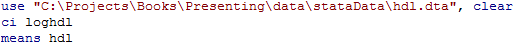﻿ Chapter 5: Introduction to presenting statistical analyses using STATA

# Chapter 5: Introduction to presenting statistical analyses using STATA

### Box 5.3 Assessing non-response bias### Box 5.3 Code### Box 5.4 Population profile from a report### Box 5.4 Code### Table 5.2 Table suitable for an oral presentation

Examples of how to do these analyses come later in the book (e.g., Figure 7.11 shows how to do a Chi-Square test and calculate relative risk for data for age; figure 10.4 shows how to do adjusted analyses using logistic regression which gives odds ratios, estimating the estimating the adjusted relative risk.

### Figure 5.5 Calculating the confidence interval of a geometric mean### Figure 5.5 Code